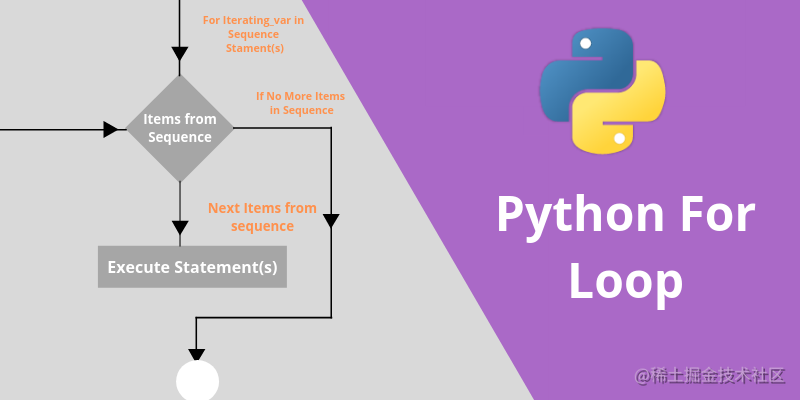# 编程中的2种循环控制指令，必不可少基础能力，看完三分钟上手

Python提供循环控制的能力，循环指令的作用是反复执行相同的任务，直到不满足循环条件为止。它也会经常出现在各大编程场景，因此也是必须掌握的。• for循环
• while循环
• break
• continue

2包

8元每包

12瓶

2.2元每瓶

20部

1988元每部

200包

90元每包

# for循环

``````data_src = [{'number':2,'price':8},{'number':12,'price':2.2},{'number':20,'price':1988}]
total_sales = 0.0
for row in data_src:
total_sales += row.number*row.price
print('the total sales of last month is %12.2f' % total_sales )

for .. in指令非常直观，它直接遍历数据集合，row代表某行数据，当遍历该集合中最后一行之后循环结束。每遍历一行都会累加这项商品的销售额。

# while循环

``````data_src = [{'number':2,'price':8},{'number':12,'price':2.2},{'number':20,'price':1988}]
total_sales = 0.0
count = len(data_src)
i = 0
while i < count:
row = data_src[i]
total_sales += row.number*row.price
i += 1
print('the total sales of last month is %12.2f' % total_sales )

while循环就不是那么直观了，首先，你需要计算出所有记录的个数，然后从0开始计数，根据计数值获取对应的记录，并将其销售额累加，直到计数值等于记录的个数。

# break

``````a = [2,5,9,10]
key = 9
count = len(a)
i = 0
found = -1
while i < count:
if a[i] == key:
found = i
break
i += 1

print(found)

# continue

``````a = [2,5,9,10]
count = len(a)
i = 0
c = 0
while i < count:
if a[i] % 2 == 1:
i += 1
continue
c += 1
i += 1

print(c)

continue指令只有在每次遍历的数值是基数时才会得到执行的机会，然而，你会发现使用continue来忽略某些迭代会比较麻烦，以上示例可以简化成以下没有continue的代码片段：

``````a = [2,5,9,10]
count = len(a)
i = 0
c = 0
while i < count:
if a[i] % 2 == 0:
c += 1
i += 1

print(c)